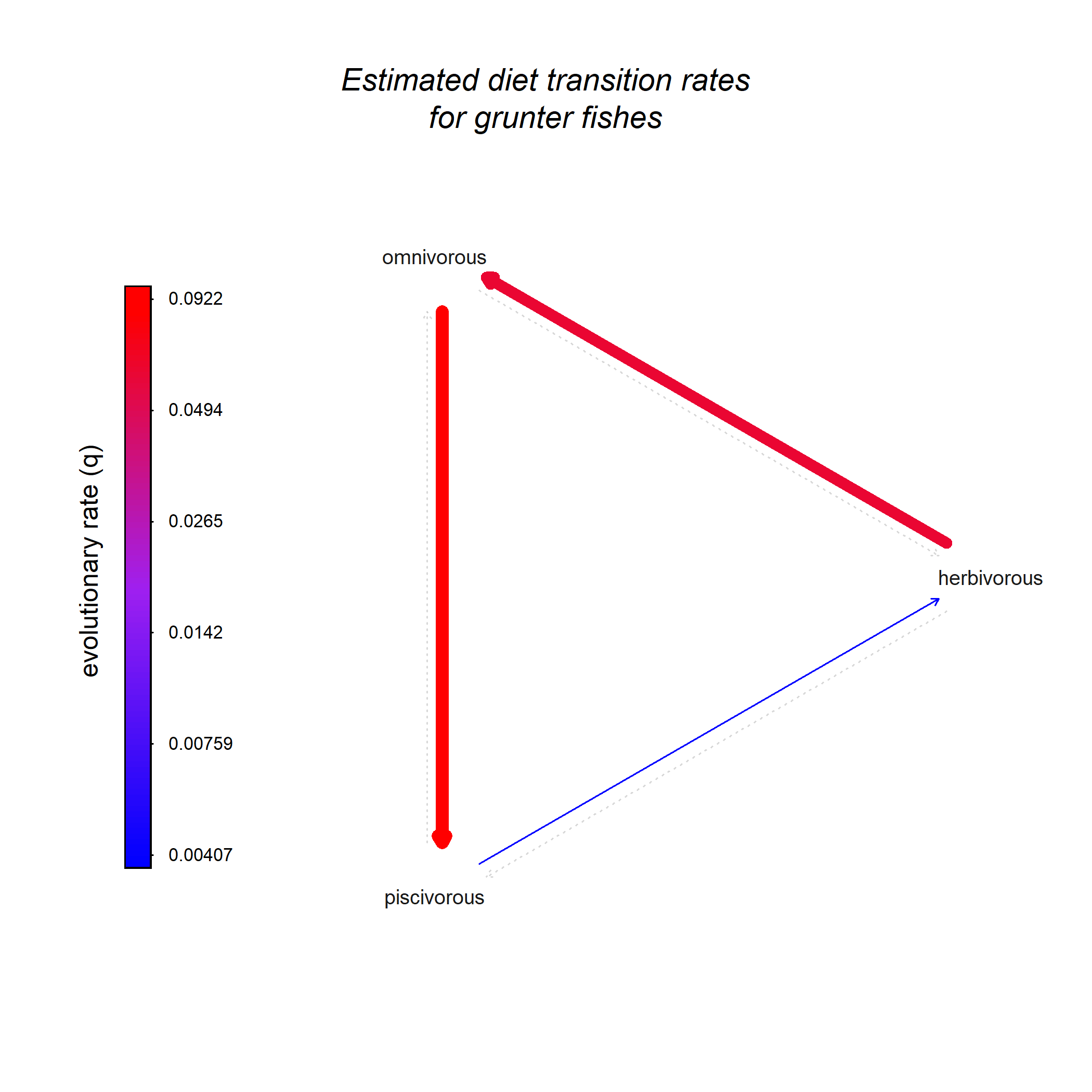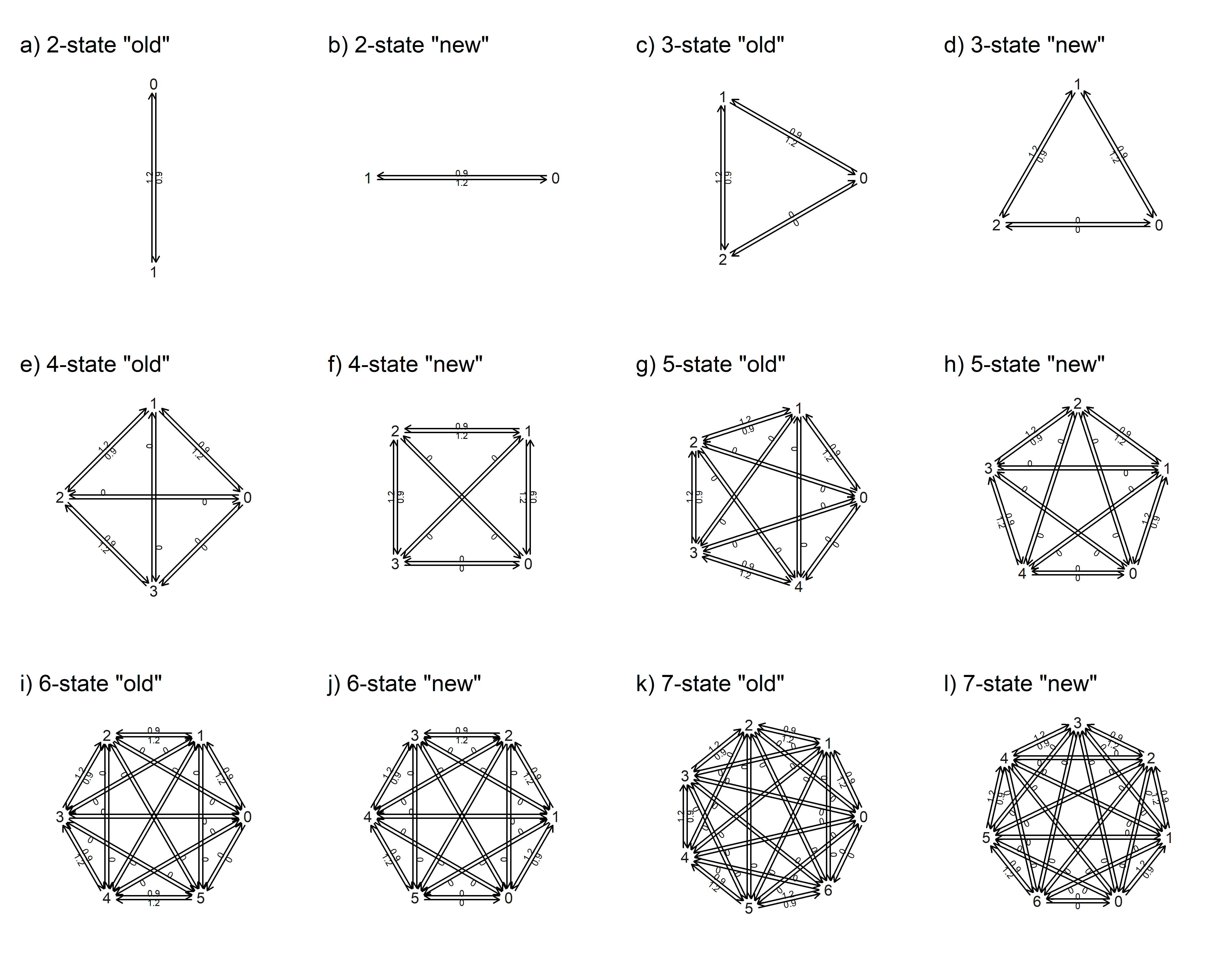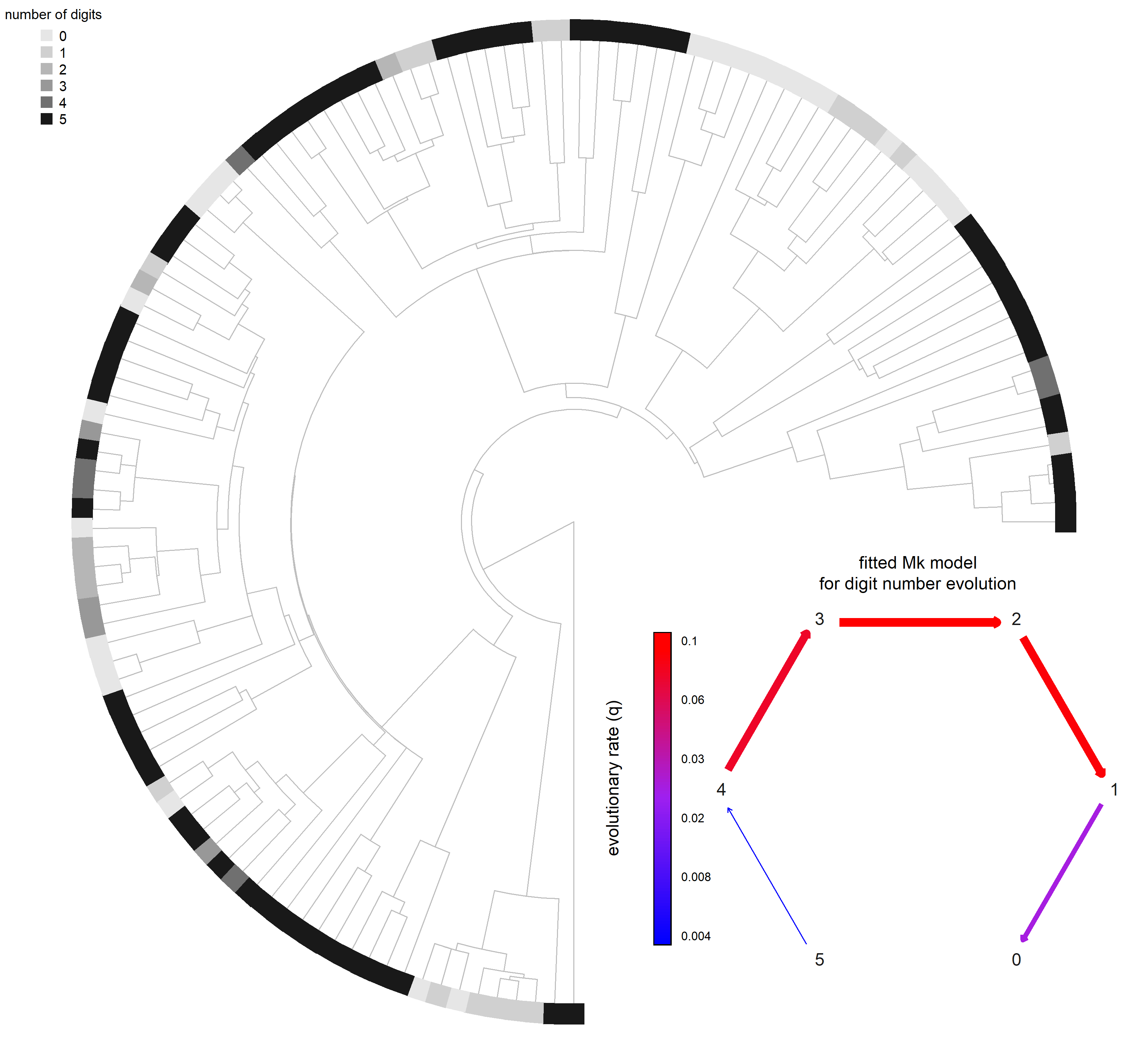## Thursday, September 8, 2022

### Updated default orientation of plotted Q matrix, for discrete character evolution (Mk models) -- and a new option to add them to an existing plot!

I recently learned on Twitter of a new paper by Kate Thomas et al. (2022) modeling the evolution of pupil shape and orientation across amphibians.

One thing that jumped out at me straight away, and reminded me of something that I've been meaning to do for a long time, was the orientation of their graphed Q matrix (and its superposition on top of the plotted tree).

Even though I have recently added a bunch of new cool functionality to the `plot.Qmatrix` method in phytools (e.g., 1, 2, 3), the default orientation of the plotted Q matrix (that is, how it is rotated around the origin) is fixed.

Since the default orientation is to start at 0 and then evenly space the trait space in an even-angle fashion, especially for low dimensional (e.g., 3 × 3) Q matrices, this can create a weird visual impression that the plotted matrix is somehow 'off-kilter'.

For example, take a look at this plotted 3 × 3 matrix from an earlier post on this blog.Here's what the update looks like – using the argument `rotate=0` to show the “old” plot orientation as well as the new default.

``````library(phytools)
``````
``````## Loading required package: ape
``````
``````## Loading required package: maps
``````
``````packageVersion("phytools")
``````
``````##  '1.2.3'
``````
``````Q2<-as.Qmatrix(matrix(c(
-0.9,0.9,
1.2,-1.2),2,2,byrow=TRUE,
dimnames=list(0:1,0:1)))
Q3<-as.Qmatrix(matrix(c(
-0.9,0.9,0.0,
1.2,-2.1,0.9,
0.0,1.2,-1.2),3,3,byrow=TRUE,
dimnames=list(0:2,0:2)))
Q4<-as.Qmatrix(matrix(c(
-0.9,0.9,0.0,0.0,
1.2,-2.1,0.9,0.0,
0.0,1.2,-2.1,0.9,
0.0,0.0,1.2,-1.2),4,4,byrow=TRUE,
dimnames=list(0:3,0:3)))
Q5<-as.Qmatrix(matrix(c(
-0.9,0.9,0.0,0.0,0.0,
1.2,-2.1,0.9,0.0,0.0,
0.0,1.2,-2.1,0.9,0.0,
0.0,0.0,1.2,-2.1,0.9,
0.0,0.0,0.0,1.2,-1.2),5,5,byrow=TRUE,
dimnames=list(0:4,0:4)))
Q6<-as.Qmatrix(matrix(c(
-0.9,0.9,0.0,0.0,0.0,0.0,
1.2,-2.1,0.9,0.0,0.0,0.0,
0.0,1.2,-2.1,0.9,0.0,0.0,
0.0,0.0,1.2,-2.1,0.9,0.0,
0.0,0.0,0.0,1.2,-2.1,0.9,
0.0,0.0,0.0,0.0,1.2,-1.2),6,6,byrow=TRUE,
dimnames=list(0:5,0:5)))
Q7<-as.Qmatrix(matrix(c(
-0.9,0.9,0.0,0.0,0.0,0.0,0.0,
1.2,-2.1,0.9,0.0,0.0,0.0,0.0,
0.0,1.2,-2.1,0.9,0.0,0.0,0.0,
0.0,0.0,1.2,-2.1,0.9,0.0,0.0,
0.0,0.0,0.0,1.2,-2.1,0.9,0.0,
0.0,0.0,0.0,0.0,1.2,-2.1,0.9,
0.0,0.0,0.0,0.0,0.0,1.2,-1.2),7,7,byrow=TRUE,
dimnames=list(0:6,0:6)))
par(mfrow=c(3,4))
plot(Q2,rotate=0)
plot(Q2)
plot(Q3,rotate=0)
plot(Q3)
plot(Q4,rotate=0)
plot(Q4)
plot(Q5,rotate=0)
plot(Q5)
plot(Q6,rotate=0)
plot(Q6)
plot(Q7,rotate=0)
plot(Q7)
``````Cool.

In addition, the tweet also shows a visual in which the Q matrix is graphed on top of the plotted tree.

I decided to add this functionality to `plot.Qmatrix` too, using the logical argument `add`.

In this example, I'm going to use a phylogeny & squamate digit number example subsampled from a dataset by Brandley et al. (2008).

In this case, rather than paste my fitted Q matrix right on top of the plotted tree, I'll graph my tree through only 75% of the space, and then add the fitted Mk model to the remaining quadrant.

The code below also includes a hacky method to visualize a discrete trait at the tips using thicken lines.

The arguments I used to adjust the position of the plotted Q matrix where `xlim` and `ylim`.

For instance, by setting the first value of `ylim` to be a relatively small (negative) number, and the latter value to be large, we get the Q matrix into the lower half (and so on). The matrix will always plot between -1 and 1 on both axes.

``````## read data & tree
chk<-geiger::name.check(sqTree,sqData)
sqTree.pruned<-drop.tip(sqTree,chk\$tree_not_data)
sqData.pruned<-sqData[!(rownames(sqData)%in%
chk\$data_not_tree),,drop=FALSE]
toes<-setNames(as.factor(sqData.pruned[,"rear.toes"]),
rownames(sqData.pruned))
## fit directional (loss only) trait evolution model
directional.model<-matrix(c(
0,0,0,0,0,0,
1,0,0,0,0,0,
0,2,0,0,0,0,
0,0,3,0,0,0,
0,0,0,4,0,0,
0,0,0,0,5,0),6,6,byrow=TRUE,
dimnames=list(0:5,0:5))
fitDirectional<-fitMk(sqTree.pruned,toes,model=directional.model,
pi="fitzjohn")
fitDirectional
``````
``````## Object of class "fitMk".
##
## Fitted (or set) value of Q:
##          0         1         2         3         4         5
## 0 0.000000  0.000000  0.000000  0.000000  0.000000  0.000000
## 1 0.024241 -0.024241  0.000000  0.000000  0.000000  0.000000
## 2 0.000000  0.103141 -0.103141  0.000000  0.000000  0.000000
## 3 0.000000  0.000000  0.109233 -0.109233  0.000000  0.000000
## 4 0.000000  0.000000  0.000000  0.083290 -0.083290  0.000000
## 5 0.000000  0.000000  0.000000  0.000000  0.004369 -0.004369
##
## Fitted (or set) value of pi:
## 0 1 2 3 4 5
## 0 0 0 0 0 1
## due to treating the root prior as (a) nuisance.
##
## Log-likelihood: -118.387049
##
## Optimization method used was "nlminb"
``````
``````## create plot
plotTree(sqTree.pruned,type="fan",ftype="off",
color="grey",lwd=1,part=0.75)
obj<-get("last_plot.phylo",envir=.PlotPhyloEnv)
n<-Ntip(sqTree.pruned)
cols<-setNames(grey.colors(n=length(levels(toes)),0.9,0.1),
levels(toes))
par(lend=3)
for(i in 1:Ntip(sqTree.pruned)){
cc<-if(obj\$xx[i]>0) 10 else -10
th<-atan(obj\$yy[i]/obj\$xx[i])
segments(obj\$xx[i],obj\$yy[i],
obj\$xx[i]+cc*cos(th),
obj\$yy[i]+cc*sin(th),
lwd=20,
col=cols[toes[sqTree.pruned\$tip.label[i]]])
}
legend("topleft",levels(toes),pch=15,col=cols,pt.cex=1.5,
cex=0.8,bty="n",title="number of digits")
text=FALSE,color=TRUE,
xlim=c(-4,0.5),ylim=c(-1,3.5),signif=1)
text(0,1.1,"fitted Mk model\nfor digit number evolution")
``````OK. It works!